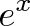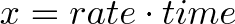# Understanding Euler’s Identity and Natural Logs

The mathematical models of plant growth are extremely complex. As farmers, We spend a good deal of time looking at growth rates of plants over time, the decay of certain elemental components in soils, rise of microbial populations, and countless other biological growth and decay models.

The most powerful tool at the farmers disposal to use in making informed decisions is the Natural log or$\displaystyle{e^x}$.

Logarithms:

The first question to ask is ‘What are logarithm’s’? Quite simply, they are the inverse of exponents.

2³ = 8 is the same as saying 2 x 2 x 2 = 8. The inverse of this would be written:  log2(8) = 3

Farmers use Logarithms all the time. An example is the pH scale. Farmers measure pH of the soil, irrigation water, and liquid fertilizers. pH is represented on a scale measuring the potential of hydrogen. 1-7 are acid and 7-14 are basic. pH is measured on a log scale. The formula for pH is as follows:

pH = – log [H3O+]

What is e ?

As you are sitting there reading this, the cells in your body are undergoing mitosis and meiosis. We all started out as a single cell, and then by the time we mature that single cell has developed into 37 trillion (or 3.7 x 10¹³)!

During mitosis, the “mother” cell generates a copy of its DNA and transfers it to the “daughter” cell. The process goes from a Single Cell to an Interphase then to two separate cells. Then those two cells repeat the process to make four, and then those four to make sixteen. The process goes on until maturity.

All continually growing rates can be measured by the base, e.  The basic equation is:$\displaystyle{e^x}$ where$\displaystyle{x = rate \cdot time}$

The base, e , is a mathematical constant that is equal to 2.718281828, and is called Euler’s identity. It should be easy to remember if you break it down into 2.7 1828 1828 The ‘1828’ repeats twice.

Where does 2.718281828 come from?

Let’s being by looking at exponential growth. Let’s assume that during mitosis, the cell divides completely in 24 hours. Therefore, with every period, we double. So for two periods, we would have the following equation:

2 x 2 = 4

3 periods or splits, would be  2³ = 8

To put this in an algebraic equation, we would write:   growth = 2x

This works well at 100% growth rate, but suppose we need 25% growth rate. We need to modify the equation to represent different percentages of growth.

growth = (1 + p)x

where p is our percentage of growth.

For the same example above, we would write

growth = (1+100%)³ = 8

Biological process do not grow to this rate though. The interphase on mitosis for example starts out slow and increases with time. Let’s look at money for a minute. If I put money in the bank, I receive a compounded interest rate. That means that my principal money is earning interest over time, but the interest money I am receiving over time is earning interest as well. That is the benefit of compounding interest rates. We now need to model our equation to account for this type of growth:

growth = (1+100%/x)x, where x is our division of time.

We all know that our compounded interest rates at the  bank are based upon time. Interest compounded bi-annually is not as good as interest compounded monthly. Let’s look at two examples with our above equation:

Bi-Annually with 100% growth on a $1 investment growth = (1+100%/2)² = 2.25 – This means that we have earned$1.25 for the initial $1.00 we put in. Let’s take our$1.00 and compound it monthly at 100% growth:

growth = (1+100%/12)¹² = 2.613  By compounding it monthly, our $1.00 has now become$1.63 in gain. We earned an additional $0.38 by compounding it more often. Let’s look at a table of our equation at various intervals of time:  x (1+100%/x)x 1 2 10 2.59374246 100 2.70481383 10,000 2.71814593 100,000 2.71826824 1,000,000 2.71828047 This is where the magic transcendental number e comes from. It is the maximum possible result when accounting for 100% growth for any one time period. How do we use Euler’s number ? Our original equation for e from above was:$\displaystyle{e^x}$ where$\displaystyle{x = rate \cdot time}$ Equation 1: Let’s write an equation for 12% growth over 1 year: (e¹²)¹ = y 1. Divide 100%/12 to yield 0.083333 2. Solve for e with the following equation 2.718281828^.083333 = 1.08690 3. = 1.0869 Therefore, we receive$0.0869 interest over 1 year in $1.00 Equation 2: Lets say we put some plant cells in a dish, and these cells divide every 24 hours. If we have 500 cells, how many will we have in 12 days? 500 cells * (e¹)¹² = 1. Multiply 1 * 12 = 12 2. Solve for e : 2.718281828^12 = 162,754.791 3. Multiply by 500 to yield 81,377,395.54 cells Natural Logarithms: Euler’s identity was the amount of continuous growth over time where the Natural Log (ln) is the amount of time needed to reach a certain level of continuous growth. The Natural Log is the inverse of Euler’s identity and it is all about time. It is abbreviated ln which stands for logarithmus naturalis. It is calculated just like a log function except the base is e. Let’s look at equation 1 from above: (e¹²)¹ = y we solved and y = 1.0869 So if we wanted to calculate how long it would take for our$1.00 to gain $0.0869 in interest, we would have the following equation: ln(1.0869) = 0.08333 then take 1/0.08333 which equals 12 periods. We now know it takes 12 periods of time to gain$0.0869 in interest.